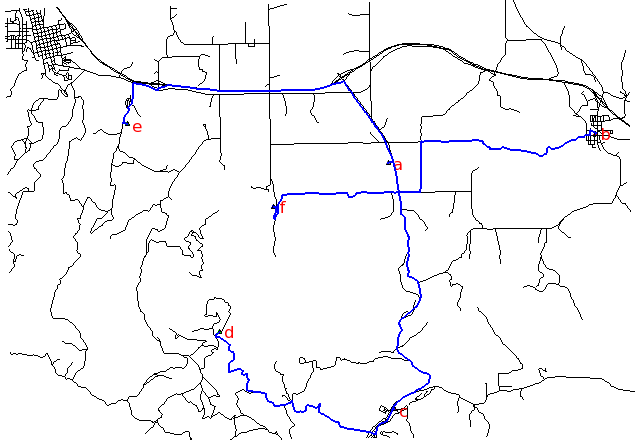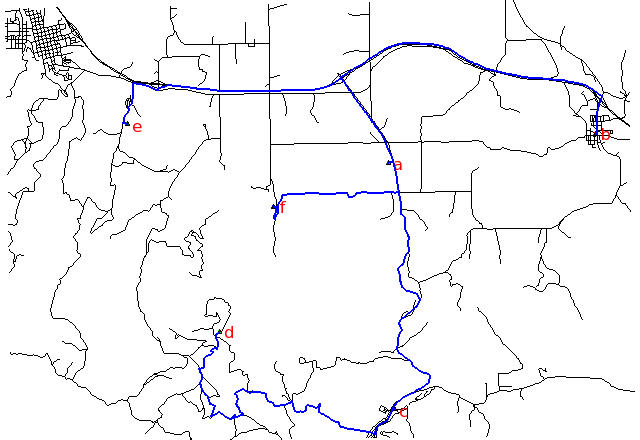Note: A new GRASS GIS stable version has been released: GRASS GIS 7.8, available here.
Updated manual page: here

## NAME

v.net.steiner - Creates Steiner tree for the network and given terminals.
Note that 'Minimum Steiner Tree' problem is NP-hard and heuristic algorithm is used in this module so the result may be sub optimal.

## KEYWORDS

vector, network, steiner tree

## SYNOPSIS

v.net.steiner
v.net.steiner --help
v.net.steiner [-g] input=name output=name [arc_type=string[,string,...]] [arc_layer=string] [node_layer=string] [acolumn=string] terminal_cats=range [npoints=integer] [--overwrite] [--help] [--verbose] [--quiet] [--ui]

### Flags:

-g
Use geodesic calculation for longitude-latitude locations
--overwrite
Allow output files to overwrite existing files
--help
Print usage summary
--verbose
Verbose module output
--quiet
Quiet module output
--ui
Force launching GUI dialog

### Parameters:

input=name [required]
Name of input vector map
Or data source for direct OGR access
output=name [required]
Name for output vector map
arc_type=string[,string,...]
Arc type
Input feature type
Options: line, boundary
Default: line,boundary
arc_layer=string
Arc layer
Vector features can have category values in different layers. This number determines which layer to use. When used with direct OGR access this is the layer name.
Default: 1
node_layer=string
Node layer (used for terminals)
Vector features can have category values in different layers. This number determines which layer to use. When used with direct OGR access this is the layer name.
Default: 2
acolumn=string
Arcs' cost column (for both directions)
terminal_cats=range [required]
Category values
Categories of points on terminals (layer is specified by nlayer)
npoints=integer
Number of Steiner points (-1 for all possible)
Default: -1

## DESCRIPTION

v.net.steiner calculates the optimal connection of nodes on a vector network.

A Steiner tree is used to calculate the minimum-cost vector network connecting some number of end nodes in a network framework. For example it could be used to find the path following a road system which will minimize the amount of fibre optic cable needed to connect a series of satellite offices.

Costs may be either line lengths, or attributes saved in a database table. These attribute values are taken as costs of whole segments, not as costs to traverse a length unit (e.g. meter) of the segment. For example, if the speed limit is 100 km / h, the cost to traverse a 10 km long road segment must be calculated as length / speed = 10 km / (100 km/h) = 0.1 h. Supported are cost assignments for both arcs and nodes. For areas, costs will be calculated along boundary lines.

Points representing nodes must be exactly on network nodes, and the input vector map needs to be prepared with v.net operation=connect.

## NOTES

Current implementation of obtaining Steiner tree is not memory efficient. An attempt to run module on a network with large number of intersections thus might result in failure to allocate memory or out of memory condition.

## EXAMPLE

Steiner tree for 6 digitized nodes (Spearfish):Fastest path, along highways:```# Spearfish

# we have 6 locations to allocate
echo "1|601653.5|4922869.2|a
2|608284|4923776.6|b
3|601845|4914981.9|c
4|596270|4917456.3|d
5|593330.8|4924096.6|e
6|598005.5|4921439.2|f" | v.in.ascii in=- cat=1 x=2 y=3 out=centers col="cat integer, \
east double precision, north double precision, label varchar(43)"

v.db.select centers
v.category centers op=report
# type       count        min        max
# point          6          1          6

# create lines map connecting points to network (on layer 2)

# set up costs as traveling time

# create unique categories for each road in layer 3

# add new table for layer 3
v.db.addtable myroads_net_time layer=3 col="cat integer,label varchar(43),length double precision,speed double precision,cost double precision"

# copy road type to layer 3
v.to.db myroads_net_time layer=3 qlayer=1 opt=query qcolumn=label columns=label

v.to.db myroads_net_time layer=3 type=line option=length col=length unit=miles

# set speed limits in miles / hour
v.db.update myroads_net_time layer=3 col=speed val="75.0" where="label='interstate'"
v.db.update myroads_net_time layer=3 col=speed val="75.0" where="label='primary highway, hard surface'"
v.db.update myroads_net_time layer=3 col=speed val="50.0" where="label='secondary highway, hard surface'"

# define traveling costs as traveling time in minutes:
v.db.update myroads_net_time layer=3 col=cost val="length / speed * 60"

# shortest path
v.net.steiner myroads_net_time arc_layer=3 node_layer=2 terminal_cats=1-6 out=mysteiner_distance

# fastest path
v.net.steiner myroads_net_time arc_layer=3 node_layer=2 acol=cost terminal_cats=1-6 out=mysteiner_time
```
To display the result, run for example:
```# display the results

# shortest path
d.mon x0
d.vect -c centers icon=basic/triangle
d.font Vera
d.vect centers col=red disp=attr attrcol=label lsize=12
d.vect mysteiner_distance col=blue width=2

# fastest path
d.mon x1
d.vect -c centers icon=basic/triangle
d.font Vera
d.vect centers col=red disp=attr attrcol=label lsize=12
d.vect mysteiner_time col=blue width=2
```

d.path, v.net, v.net.alloc, v.net.iso, v.net.path, v.net.salesman